Courses

# Orbitals , Wave Functions and Quantum Numbers Class 11 Notes | EduRev

## Class 11 : Orbitals , Wave Functions and Quantum Numbers Class 11 Notes | EduRev

The document Orbitals , Wave Functions and Quantum Numbers Class 11 Notes | EduRev is a part of the Class 11 Course Chemistry for JEE.
All you need of Class 11 at this link: Class 11

Quantum Numbers:

In the solution to the Schrodinger equation for the hydrogen atom, three quantum numbers arise from the space geometry of the solution and a fourth arises from electron spin. No two electrons can have an identical set of quantum numbers according to the Pauli exclusion principle , so the quantum numbers set limits on the number of electrons which can occupy a given state and therefore give insight into the building up of the periodic table of the elements.(1) Principal Quantum No. (n) :

The principal quantum number or total quantum number n arises from the solution of the radial part of the Schrodinger equation for the hydrogen atom. The bound state energies of the electron in the hydrogen atom are given by En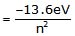(i) Permissible values of n : all integers from 1 to ∞ (infinity).
(ii) This no. identifies shell in an atom.
(iii) It gives an idea of average distance `R' [size of any orbital from the nucleus higher the value of, n, higher will be the average distance and hence greater will be the size].
(iv) It gives idea of energy of electron upto some extent. Higher the value of n, higher will be the energy (if `l' is constant). For example order of energy 4s > 3s > 2s > 1s.
(v) It gives value of total no. of orbitals present in any shell and that is equal to n2.
(vi) It gives value of total no. of electrons which may he present in the given shell = 2n2.
(vii) It gives value of angular momentum of electron i.e.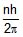(viii) It give variation of radial probability distribution.(2) Azimuthal Quantum No. or Angular momentum Quantum no. (lPermissible values of l is 0 to (n -1). i.e. value of l is restricted by n.

n = 1, = 0

n = 2, = 0, 1. ; i.e. 2 values of .

n = 3, = 0, 1, 2. i.e. 3 values of .

No. of values of `l' is equal to `n' principal quantum number

It identifies sub shell in an atom

The value of `l' gives name of sub-shell and shape of orbital.

 ℓ Notation Name Shape o s Sharp Spherical 1 P Principal dumbell shaped 2 d diffuse double dumbell 3 f fundamental complex

(3) Magnetic Quantum Number (m) The direct implication of this quantum number is that the z-component of angular momentum is quantized according to Lz = mlh/2π

It is called the magnetic quantum number because the application of an external magnetic field causes a splitting of spectral lines called the Zeeman effect. The different orientations of orbital angular momentum represented by the magnetic quantum number can be visualized in terms of a vector model.(4) Spin quantum number (s) :

An electron, besides charge and mass, has also spin angular momentum commonly called spin. The spin angular momentum of the electron is constant and cannot be changed.

An electron spin s = 1/2 is an intrinsic property of electrons. Electrons have intrinsic angular momentum characterized by quantum number 1/2. In the pattern of other quantized angular momenta, this gives total angular momentum

The resulting fine structure which is observed corresponds to two possibilities for the z-component of the angular momentum.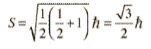This causes an energy splitting because of the magnetic moment of the electron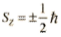Orbitals:Orbitals are defined as that region or zone in the space where probability of finding electrons is possible (more than 90%). In an atom large no. of permissible orbitals are present.

These orbitals are designated by a set of 3 quantum number (n, l, m) which arise as a natural consequence in the solution of Schrodinger equation i.e. the values of 3 quantum numbers are restricted by the solution of Schrodinger equation.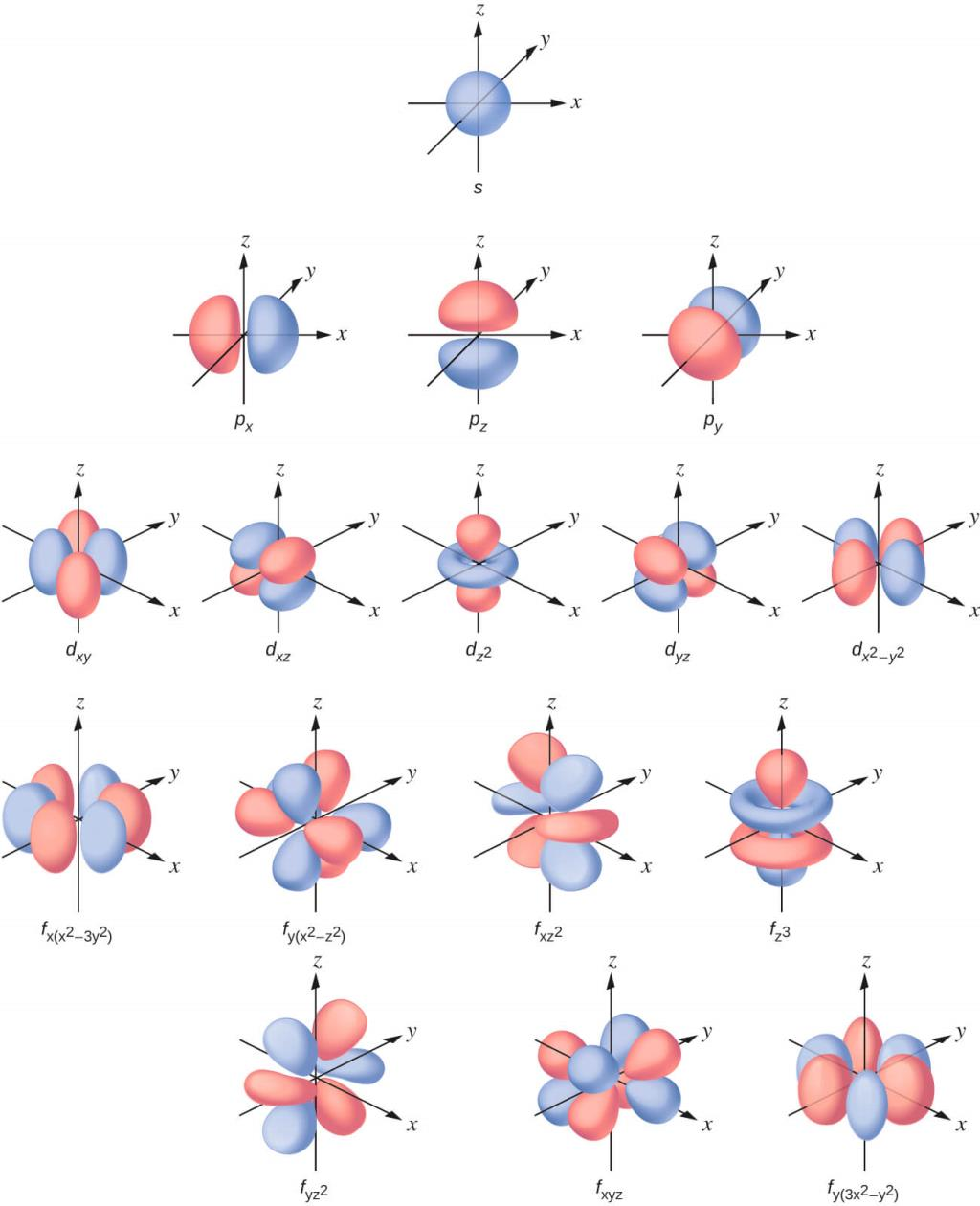Fig: Atomic orbitals.

The Postulates of Quantum Mechanics:

1. Associated with any particle moving in a conservative field of force is a wave function which determines everything that can be known about the system.
2. With every physical observable q there is associated an operator Q, which when operating upon the wave function associated with a definite value of that observable will yield that value times the wave function.
3. Any operator Q associated with a physically measurable property q will be Hermitian.
4. The set of eigen functions of operator Q will form a complete set of linearly independent functions.
5. For a system described by a given wave function, the expectation value of any property q can be found by performing the expectation value integral with respect to that wave function.
6. The time evolution of the wave function is given by the time dependent Schrodinger equation.

The Wave function Postulate:

It is one of the postulates of quantum mechanics that for a physical system consisting of a particle there is an associated wave function.
This wave function determines everything that can be known about the system. The wave function is assumed here to be a single-valued function of position and time, since that is sufficient to guarantee an unambiguous value of probability of finding the particle at a particular position and time.
The wave function may be a complex function, since it is its product with its complex conjugate which specifies the real physical probability of finding the particle in a particular state.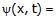single-valued probability amplitude at (x, t)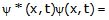probability of finding particle at x at time t provided the wave function is normalized.

Shapes of Atomic Orbitals.

The problems associated with the representations of the variations of |Y|2 in space have been circumvented by the following two approaches.

(a) Charge cloud diagrams (b) Boundary surface diagrams.

(A) Charge cloud diagrams

In this approach, the probability density |Y|2 is shown as a collection of dots such that the density of dots in any region represents the electron probability density in that region. Fig. shows such plots for some orbitals. These give some ideal about the shapes of the orbitals.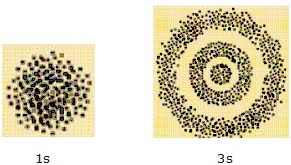Fig: orbital shapes.

(B) Boundary surface diagrams:

In these diagrams, the shape of an orbital is defined as a surface of constant probability density that encloses some large fraction (say 90 %) of the probability of finding the electron. The probability density is |Y|2. When |Y|is constant, so is |Y|. Hence |Y| is constant on the surface for an s orbital (l = 0) has the shape of a spherical shell centered on the nucleus, fig. For each value of n, there is one s orbital. As n increases, there are (n-1) concentric spherical shells like the successive layers in an onion.

Shapes of Atomic Orbitals:

The shapes of the orbitals are
s-spherical, p-dumb bell, d-double-dumb-bell, f-Diffused

These orbitals combine to form subshell.
(i) s-subshell will have only one spherical orbital.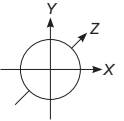(ii) p-subshell has three orbitals (px, py, pz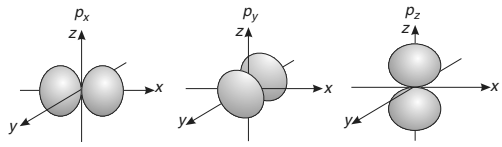(iii) d-subshell has five orbitals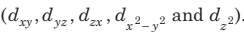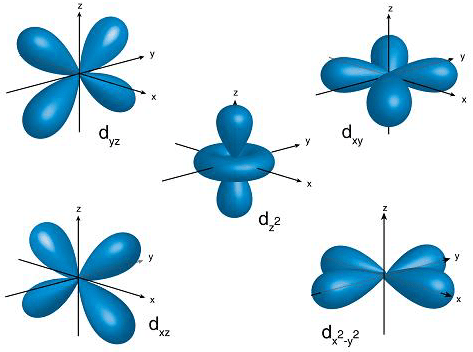Offer running on EduRev: Apply code STAYHOME200 to get INR 200 off on our premium plan EduRev Infinity!

## Chemistry for JEE

223 videos|452 docs|334 tests

,

,

,

,

,

,

,

,

,

,

,

,

,

,

,

,

,

,

,

,

,

,

,

,

;